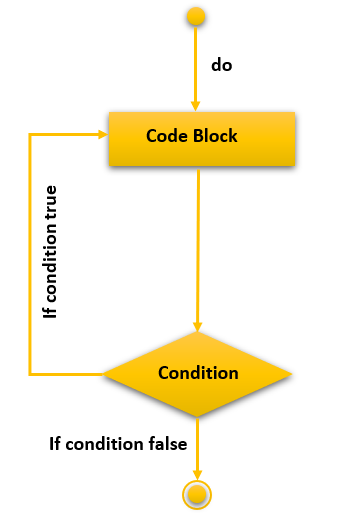# C Do While Loop

In this tutorial, you will learn about the do-while loop in the C programming language with the help of examples.

In C, do-while Loops are used when you do not know how many times the loop will execute but you need it to execute for a minimum of once. It is a type of while loop but the statements are executed first and then the condition is tested. For and While are entry controlled loops but do-while is an exit controlled loop.

## C Do While Loop Syntax

The syntax for the do-while loop is as follows:

```Initialization of the expression
do
{
// loop body which will be executed at least once.
update_expression;
}
while (test or condition expression);```

On breaking down the different parts of a for loop we will get the following :

• Initialization Expression: This expression is used to initialize the do-while loop with an initial value by either declaring and initializing a variable there or only by initializing an existing variable at that point. It is declared outside the body of the loop. For example, `int i=6;` or `j=k;` // Provided both `j` and `k` have been declared and `k` has been initialized.
• Update Expression: This expression will get executed before the statements in the do-while loop have been executed and before the loop condition is evaluated. It will execute as many times as the do-while loop statement will get executed and it can be mentioned anywhere in the do-while block. Eg. `i++;`
• Test or Exit Condition: This expression will keep on getting executed until the condition is false and then the program flow will move out of the do-while loop. If the condition is true, it will move into the block and execute the statements inside the do-while loop. Even if the condition is false from the beginning, the block will get executed at least once. Eg. `i<10;`

## Flowchart of Do While Loop in C## The Structure of Do-While Loop Execution

The “Do-While Loop” execution follows the following pattern of code execution which are as follows:

1. Execution begins by initialization
2. The loop block and the update statement are executed.
3. After the update, the control moves to the condition statement in the next step
4. The execution moves onto the condition of the do-while loop.
1. If the condition is true, the flow moves onto the body.
2. If the condition is false, the flow moves to the outside of the body of the loop.
5. The above statements from steps 4 to 2 are repeated till the condition is false and it exits from the block.

## C Do While Loop Example

```#include<stdio.h>
int main(){
// Initialization of the expression
int i = 2;
do {
// Loop body statement
printf("Hello World\n");
// Update the expression
i++;
}while (i < 1); // Condition expression. There is a semi-colon after while.
return 0;
}```

Hello World

## Program Flow

1. Program starts.
2. `i` is initialized to 2.
3. Execution enters the loop.
a) “Hello World” gets printed 1st time.
b) Updation is done. Now `i = 2`.
4. Condition is checked. 2 < 2 yields false.
5. The flow goes outside the loop.

### Example 2: Do While Loop

```#include<stdio.h>
int main(){
// Initialization expression
int i = 1;
do {
// Loop body
printf("%d\n",i);
// Update expression
i++;
}while (i <= 5);
return 0;
}```

1
2
3
4
5

## Nested Do While Loop

You can nest one do-while loop inside another by simply writing the second do-while loop code inside the first do-while loop block. For example, the following code will explain how a nested do-while loop works.

```#include<stdio.h>
int main(){
int j,i=1;
do {
j=i;
do {
printf("%d\t",j);
j++;
} while ( j<=5 );
printf("\n");
i++;
} while ( i<=5 );
return 0;
}```

1 2 3 4 5
2 3 4 5
3 4 5
4 5
5

### Infinite Do-While Loop

An infinite Do-While loop can be due to a logical error or can be deliberately placed for a particular reason like waiting for the program to receive an input from a source and then continue execution. It can be achieved by either removing the exit condition or by not providing an update statement or even both.

```#include<stdio.h>
int main(){
// Initialization expression
int i = 1;
do { // No update statement
// Loop body
printf("Hello World\n");
}while (i <= 5);
return 0;
}```

Hello World
Hello World
Hello World
Ctrl + C

### Example 2: Do While Loop

```#include<stdio.h>
int main(){
// Initialization expression
int i = 1;
do { // Condition is always true
// Loop body
printf("Hello World\n");
i++;
} while ( i>0 );
return 0;
}```

Hello World
Hello World
Hello World
Ctrl + C